### vector<int>& nums1 函数参数是什么意思 [问题点数：20分，结帖人writeeee]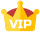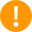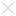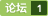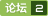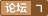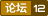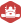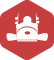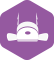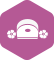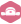2012年 总版技术专家分年内排行榜第七
1.两数只和
#include &lt;vector&gt; using std::vector; class Solution { public: vector&lt;int&gt; twoSum(vector&lt;int&gt; &amp;nums, int target) { vector&lt;int&gt; two; for (int i = 0; ...

NSCache
http://nshipster.cn/nscache/可怜的 NSCache 一直处于 NSMutableDictionary 的阴影之下。就好像没有人知道它提供了垃圾处理的功能，而开发者们却费劲力气地去自己实现它。没错，NSCache 基本上就是一个会自动移除对象来释放内存的 NSMutableDictionary。无需响应内存警告或者使用计时器来清除缓存。唯一的不同之处是键对象不会像 NSM
vector::iterator it
vector vect; vector::iterator  it = vect.begin();
vector《int*》和vector《struct *》
vector&amp;lt;int *&amp;gt;和vector&amp;lt;struct *&amp;gt; 题目是这个，居然作为标题发布不了，提示我输入有误！！！！//前言：在看VINS源码的时候发现的， 源码里面是这样的std::vector&amp;lt;ResidualBlockInfo *&amp;gt; factors;  //这里的ResidualBlockInfo是这样定义的struct ResidualBlockInf...

Vector基础知识
Vector的概念 vector跟数学上的向量并没有任何关系，仅仅是一个名字，千万别翻译成中文，最好叫它动态数组。 Vector的属性 vector优异性能就是配置比其所容纳的元素所需更多的内存，一般在使用vector之前，就先预留足够空间，以避免二次分配，这样可以使vector的性能达到最佳。因此元素个数_Count是个远比元素值 _Val重要的参数，因此当构造一个vector时，首要参数...

return vector(); 是怎么发挥作用的？

C++ vector 类学习笔记
vector简介       vector是STL中最常见的容器，它是一种顺序容器，支持随机访问。vector是一块连续分配的内存，从数据安排的角度来讲，和数组极其相似，不同的地方就是：数组是静态分配空间，一旦分配了空间的大小，就不可再改变了；而vector是动态分配空间，随着元
1. Two Sum（重要！）
Given an array of integers, return indices of the two numbers such that they add up to a specific target. You may assume that each input would have exactly one solution. Example: Given nums =
C++（笔记）容器(vector)作为函数参数如何传参

C++ vector类型 简单使用迭代器出现异常 不知道是什么原因

vector〈int〉实现堆栈
KSum问题
KSum是Leetcode上的一系列问题，本质上还是hash问题。现在从最基本的2Sum开始拓展到KSum. Two Sum  Total Accepted: 112324 Total Submissions: 635568 Given an array of integers, find two numbers such that they add up to a
vector< pair > >v ;
vector< pair > >v ; vector temp ; temp.push_back(1); v.push_back( make_pair<in
c++ vector初始化

http://www.aqee.net/this-is-how-i-work/ 大家好！我是Semih Yağcıoğlu. 我是一个程序员。我生活中土耳其首都安卡拉。我喜欢编程，挑战难题永远是让我兴奋的事情。 我会写一些我感觉有趣的东西。 这是长话短说——来和我喝一杯咖啡，我们可以细聊。 这些年来我养成了一些生活及工作上的习惯。我想这些习

vector 转换为 int*
// libvtftp.cpp : Defines the entry point for the console application. // #include "stdafx.h" #include #include void ShowIntArray(int* p, int nSize) { if (NULL == p || nSize <= 0) { return; }
NO.1两数之和问题

vector与vector的区别

LeetCode-TwoSum

D:\\ApiTest\\xml\\英文1 这个文件下是有文件的
C++中vector（向量）基本使用方法

LeetCode第一题：两数之和-C++

vector< vector > iv这种定义是什么含义？
vector< vector > iv这种定义书上说是正确的，但是我无法通过编译，它代表什么含义呢如下test代码为何无法通过编译呢？ #include #inclu

vector
vector的介绍及使用 vector的深度剖析及模拟实现 1.vector的介绍及使用 1.1 vector的介绍 vector是表示可变大小数组的序列容器。 就像数组一样，vector也采用的连续存储空间来存储元素。也就是意味着可以采用下标对vector的元素进行访问，和数组一样高效。但是又不像数组，它的大小是可以动态改变的，而且它的大小会被容器自动处理。 本质讲，vector使用动态分...

DB2 左连接的例子

C++中vector&v这种写法是什么意思啊?

vector a 这种写法对吗？如何解释？
vector a 这种 vector 的数组

jqGrid3.6.2版本 jqGrid中的常用编辑功能就在在定义editurl属性 例如editurl:'tt.jsp', 在实际开发工作中，我们经常需要对editurl的返回值进行判定（例如提交出错/提交数据重复这样的提示判定等等）并提示不同的信息。 如何来实现呢？ 从网上查询得知关键是重写navgrid的editOptions 例如： var fn_editSubmit=fun...
vector a的解释

DB2内连接查询和外连接查询
DB2内连接查询返回连接表中符合连接条件和查询条件的数据行，下面就为你详细介绍DB2内连接查询的方法，供您参考学习。 　　DB2内连接查询(INNER JOIN)： 　　DB2内连接查询有两种，显式的和隐式的，返回连接表中符合连接条件和查询条件的数据行。(所谓的链接表就是数据库在做查询形成的中间表)。 　　例如：下面的语句3和语句4的结果是相同的。 　　语句

一、BasePath &amp;lt;%String path = request.getContextPath();String basePath = request.getScheme()+&quot;://&quot;+request.getServerName()+&quot;:&quot;+request.getServerPort()+path+&quot;/&quot;;%&amp;gt;   看看上面的代码，我们来了解一下它具体的意思，  ...
vector::size_type什么意思
vector::size_type是什么意思
[转载]我再也不想在任何头文件中看到“using namespace xxx;”了

http://www.cocoachina.com/ask/questions/show/107454/为什么要复制对象？能举个例子么？ 这个翻译，有点误导啊。 我觉得原文应该是这个意思： 为什么使用copy，而不是使用retain。   简单点哈，比如 有一个 NSString *string1 = xxxx ; 另外一个是NSString *string2.
python 小白求助 这个错误是什么意思呢？

vs2015中Microsoft.VsHub.Server.HttpHost.exe进程干什么用的？

XML文件内容如下： whg whg@263.net <ma
WIN9X下内核线程注入及进程不死术，彻底保护防火墙

_=_ _=_ Part 001 of 001 of file x _=_ begin 666 x M4\$L#!!0``P`(`/&,52]LIZ<A\$``/P_```*````475A;G1I9GD

vector 详细用法 C++

supplied argument is not a valid stream resource 这个又是什么意思呢？

vector错误问题
#include #include #define MAX 10 using namespace std; int n; //È«ÅÅÁÐ1~n vector<in
leetcode 1. 两数之和 暴力穷举 排序 哈希表 时间比较

TreeNode.Tag到底是什么意思？

Two Sum
class Solution { public: vector&amp;lt;int&amp;gt; twoSum(vector&amp;lt;int&amp;gt;&amp;amp; nums, int target) { vector&amp;lt;int&amp;gt; res; int i , j; for(i = 0; i &amp;lt; nums.size(); i ++){ ...

my_leetcode
1.Two Sum class Solution { public: vector&amp;lt;int&amp;gt; twoSum(vector&amp;lt;int&amp;gt;&amp;amp; nums, int target) { vector&amp;lt;int&amp;gt;ans; int length=nums.size(),i,j; int mark...
vector里能否用引用?
class A { //省略 }; class B { vector jip; }; 大致这个样子
c++中vector和vector的用法比较

LeetCode 1. Two Sum 解题报告

vector的初始化： vector&amp;lt;T&amp;gt; v(n,i)形式，v包含n个值为i的元素:       vector&amp;lt;int&amp;gt; ivec(10,0); vector&amp;lt;T&amp;gt; v(v1)形式，v是v1的一个副本:       vector&amp;lt;int&amp;gt; ivec1(ivec); vector&amp;lt;T&amp;gt; v(n)形式，v包含n个值初始化的元素:    ...
【C++】vector v; sizeof(v)的值到底代表什么？
vector v; 问：v的大小是多少？ sizeof(v)应该就是v的大小吧？ 可是在VS中跑出来的结果有点疑问 vector v1{1,1,1}; vector v2
vector详解

LeetCode第1题
vector twoSum(vector& nums, int target) { vectorb = nums; sort(nums.begin(), nums.end()); int i = 0, j = nums.size() - 1; while (1){ if (nums[i] + nums[j] i++; else if (nums[i] + nums[j] > tar
LeetCode001

LeetCode.1求两数之和

vector >array 理解

leetcode1 Two Sum题解

Leetcode 第 1 题(Two Sum)
Leetcode 第一题昨天去逛了逛这个网站，感觉挺有意思。准备有空的时候就做一两道。第一道题目是这样的： Given an array of integers, return indices of the two numbers such that they add up to a specific target. You may assume that each input wou

LeetCode之Two Sum
class Solution { public: vector twoSum(vector& nums, int target) { unordered_map m;//使用map，key和value对应 for (int i = 0; i < nums.size(); ++i) { if (m.count(t
leetcode

LeetCode算法详解（一）--Two Sum

LeetCode题目解析（一）：1、two sum
1、两数和 问题描述： 给定一个整数数组与一个目标数，从数组中找出两个数使得这两个数之和为目标数，返回他们的下标（假定答案唯一且一个数只能被使用一次） 问题分析： 这题表面上看需要遍历数组中两两之和，并与目标进行比较，需要O(n²)的时间复杂度。但我们只需要利用哈希表就可以将时间复杂度降到O(n)。 首先定义一个哈希表（即c++的map），把值作为key，把下标作为value，遍历数组，...
Two sum 数组中两个数字的和为指定的target值
Given an array of integers, return indices of the two numbers such that they add up to a specific target.You may assume that each input would have exactly one solution, and you may not use the same el...
Leetcode Two sum问题
C++方法一：通过缩小内层遍历的次数，减少无用的重复查找class Solution { public:     vector&amp;lt;int&amp;gt; twoSum(vector&amp;lt;int&amp;gt;&amp;amp; nums, int target) {         vector&amp;lt;int&amp;gt; res(2);         for(int i=0;i&amp;lt;nums.size()-1;i+...
leetcode-两数之和-C++

[C++]两数之和

C++学习（0）之vector
C++小白在学习leetcode时遇到的问题 vector是什么？ vector是一种包含相同数据类型的对象的一种容器（或集合，是一种标准库）。vector对象能高效增长。 1、vector创建 vector v1（v1为对象名称）。 例如：vector v1；  vector V1；  vector V1；//假设Rect是一种定义的结构体。 2、vector相关函数 可以在v
C++中 vector>

vector< vector > vvi 是否可行？

java开发的台球游戏源码下载